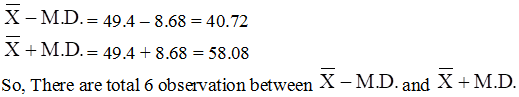# RD Sharma Solutions for Class 11 Chapter 32 - Statistics Exercise 32.1

In this exercise, we shall discuss measures of dispersion, range (the range is the difference between two extreme observations of the distribution) and mean deviation. Students who wish to increase their knowledge about the concepts and build strong command over the subject can refer to RD Sharma Class 11 Maths Solutions. Subject experts have formulated the solutions in a simple and understandable language to meet the requirements of students and help them secure good marks in the board exams. Students are advised to practice regularly, so that it helps them to come out with flying colours in their board exams. Here, the solutions to this exercise are provided in pdf format, which can be downloaded easily from the links given below.

## Download the pdf of RD Sharma Solutions for Class 11 Maths Exercise 32.1 Chapter 32 – Statistics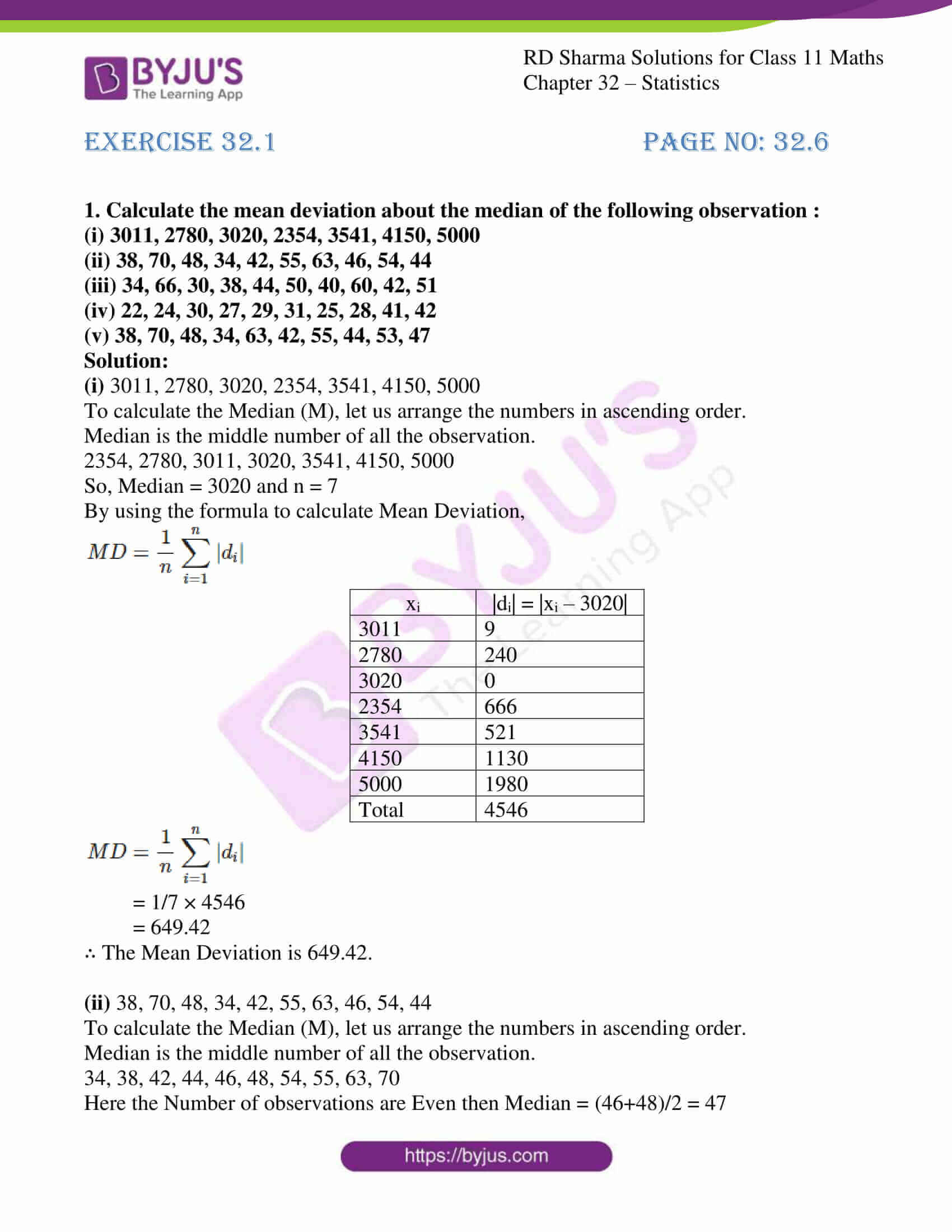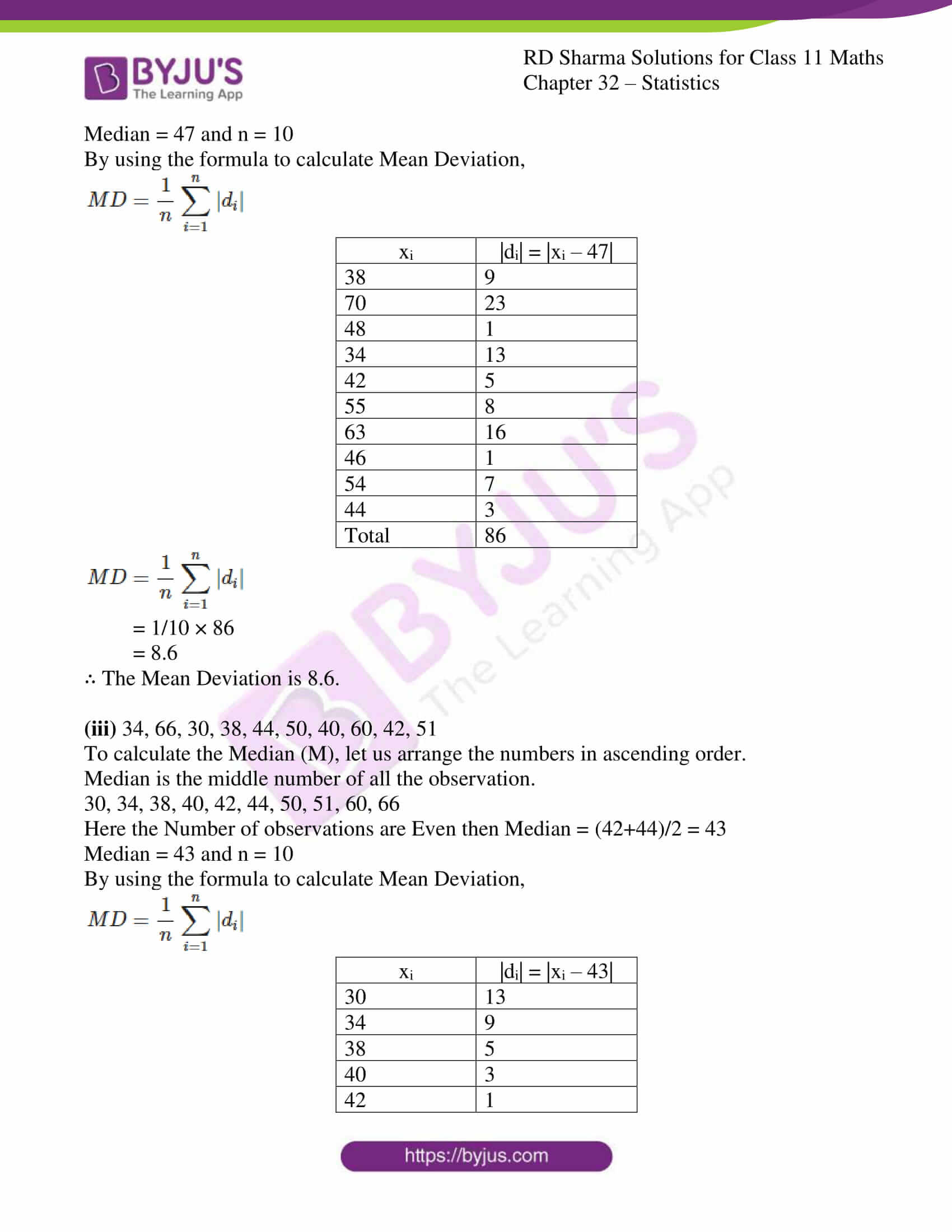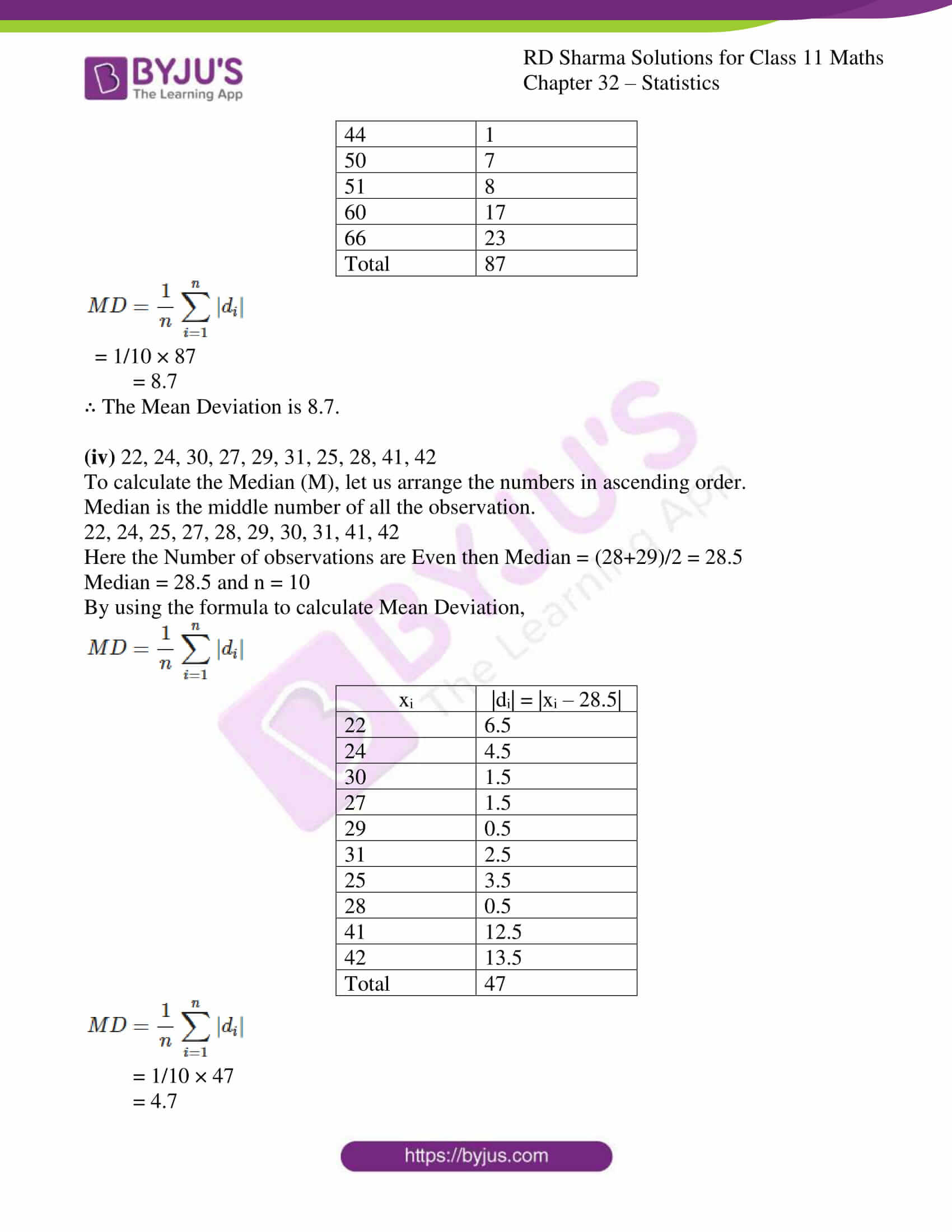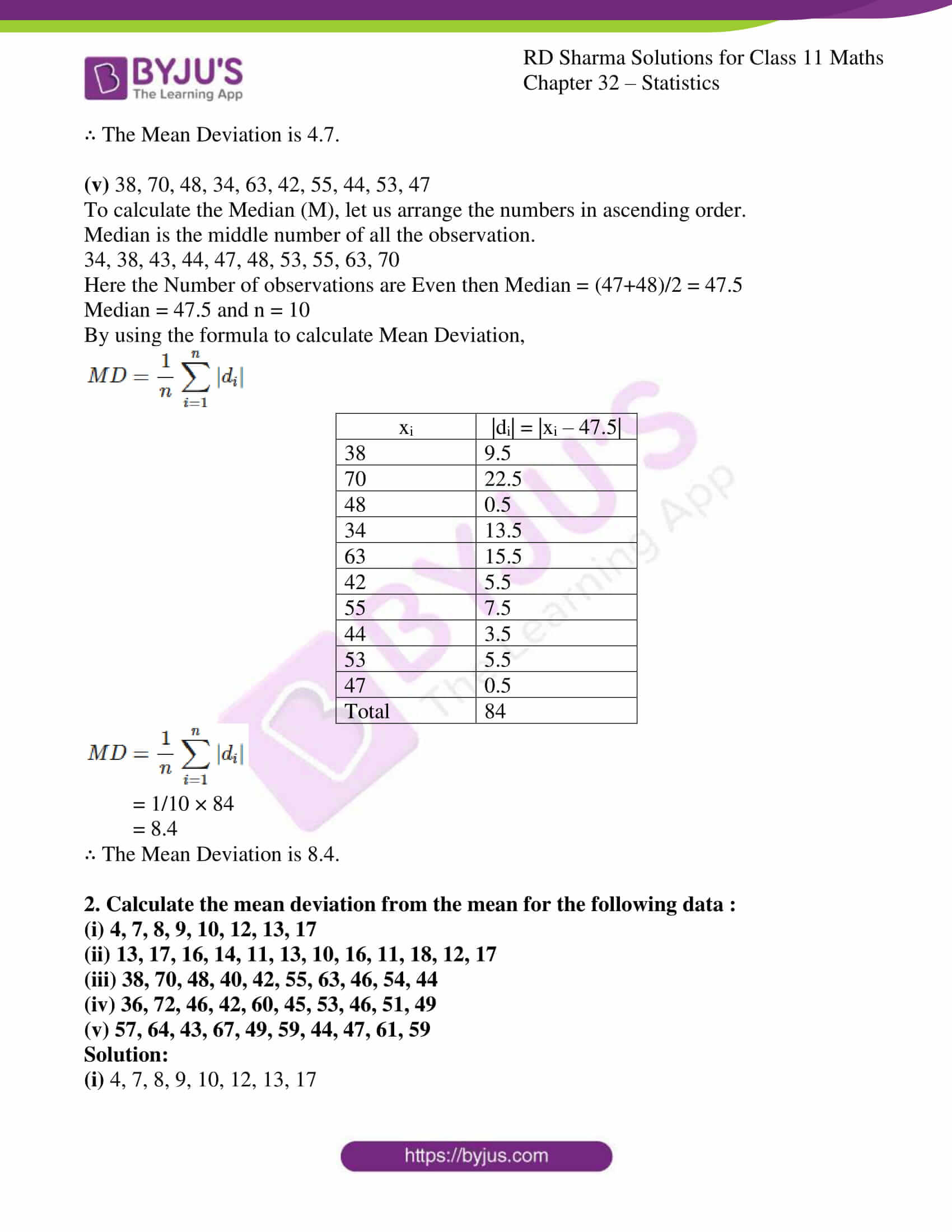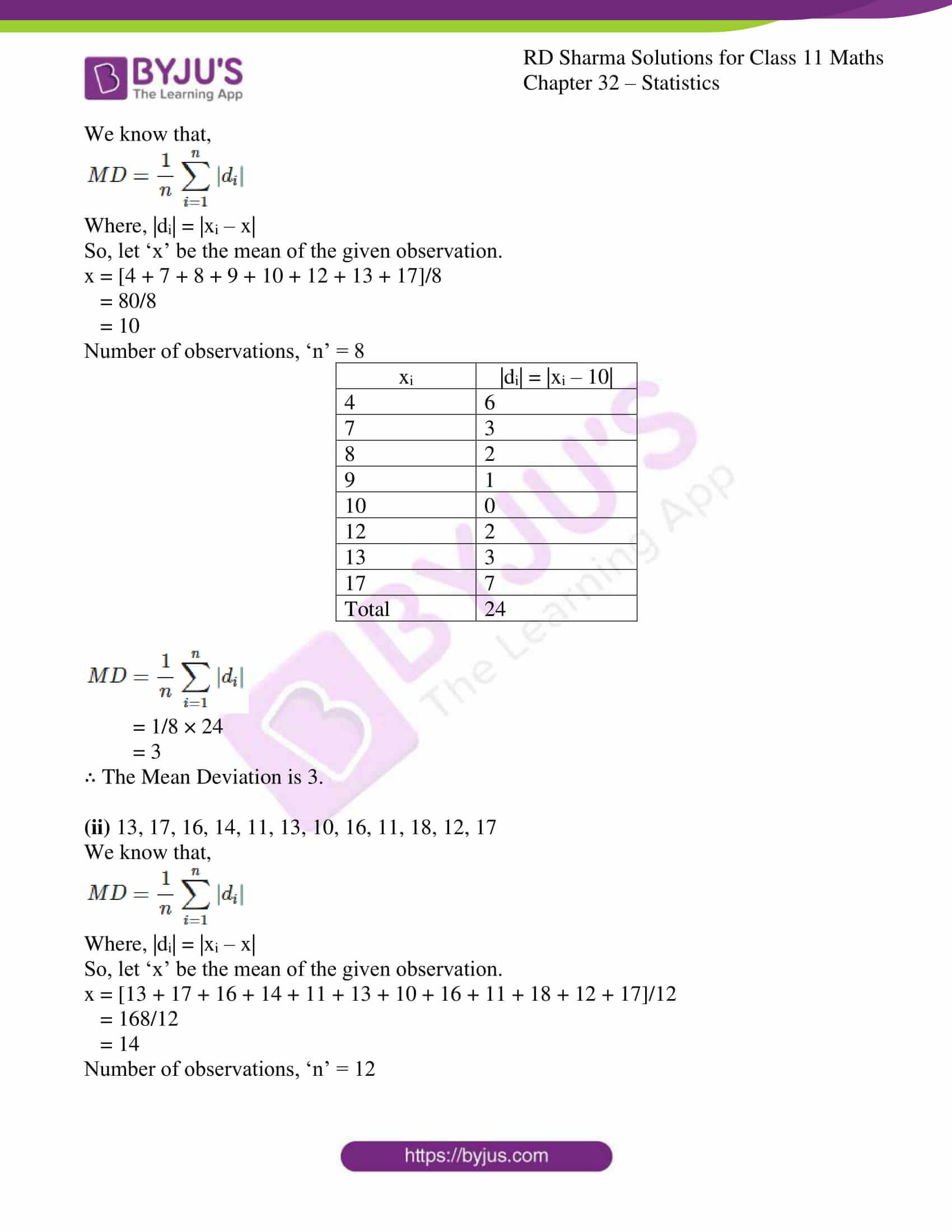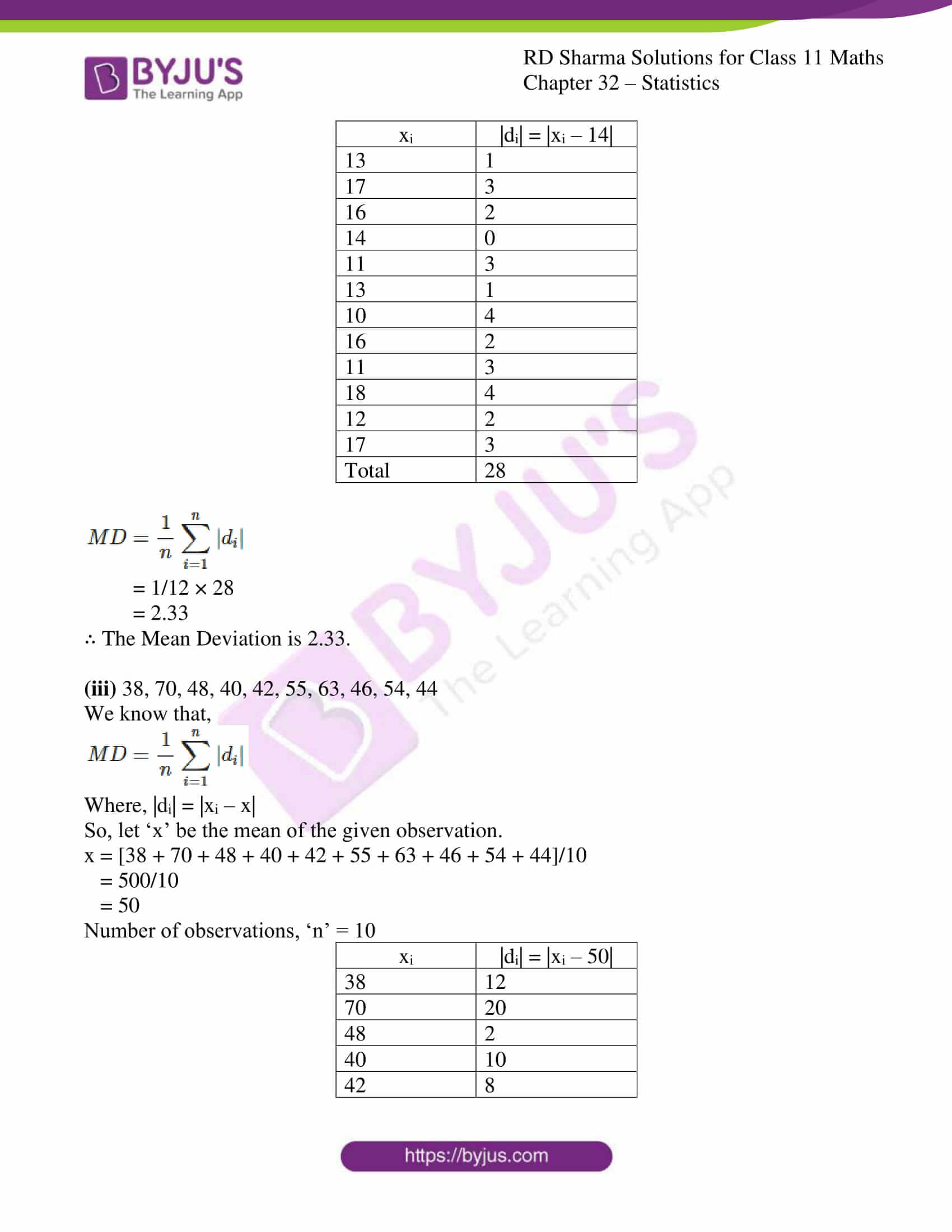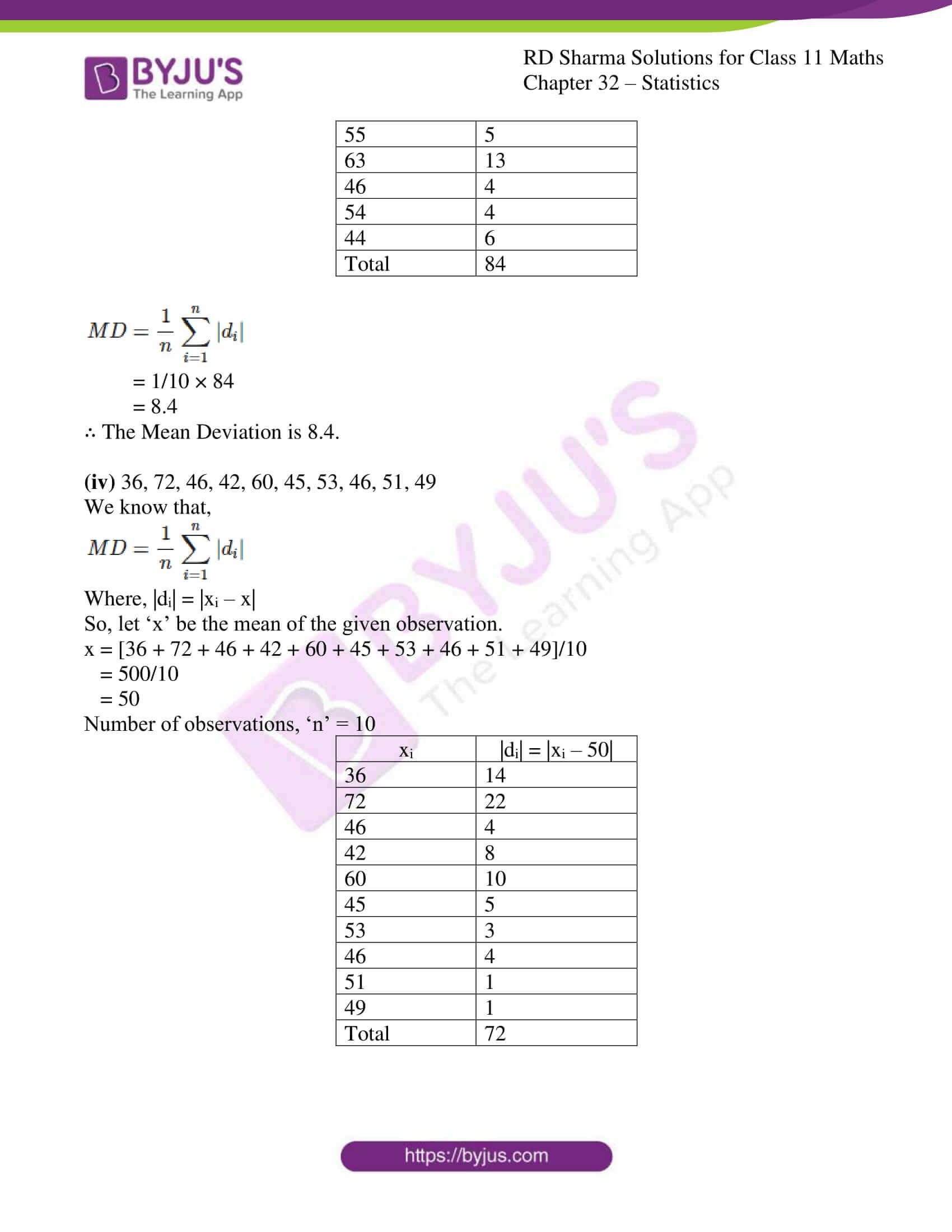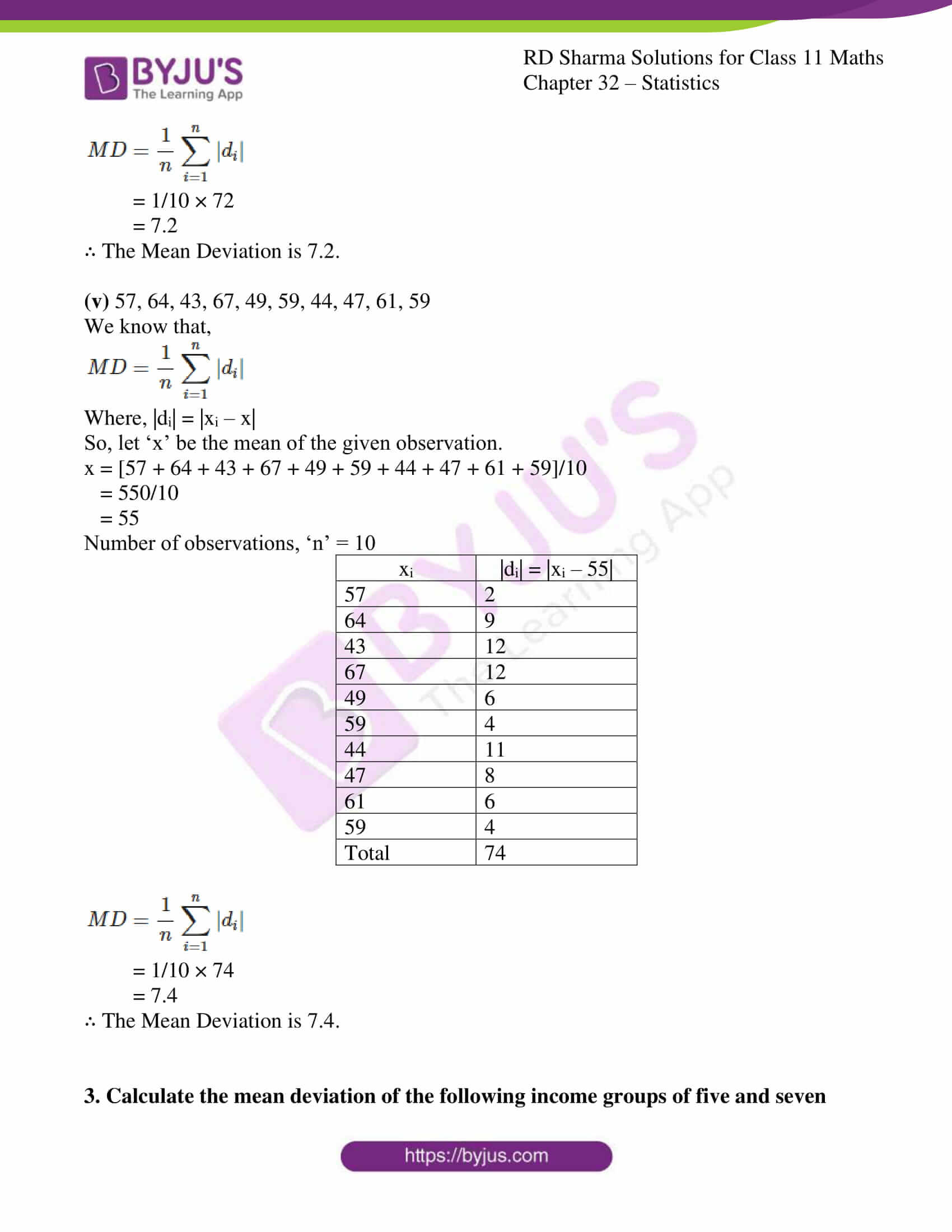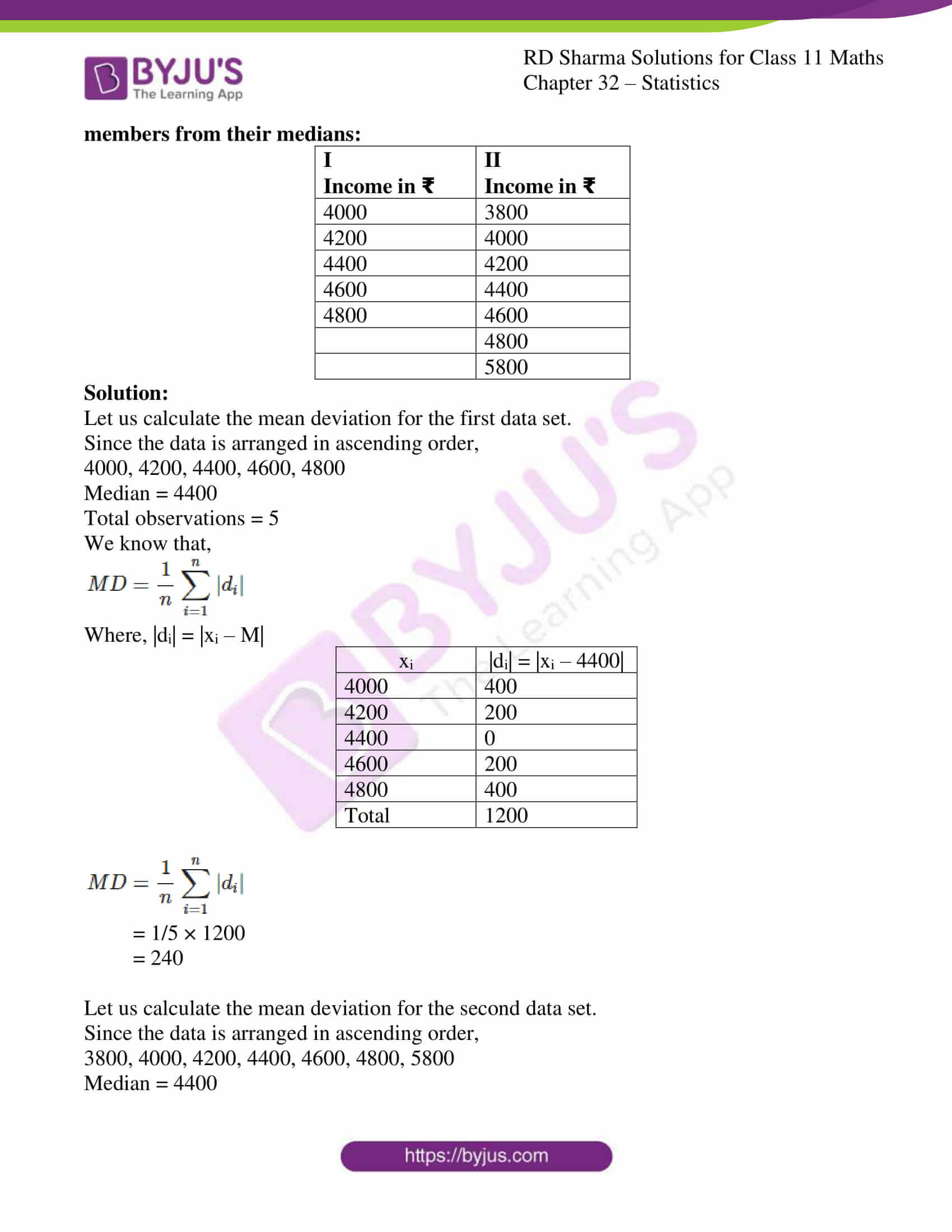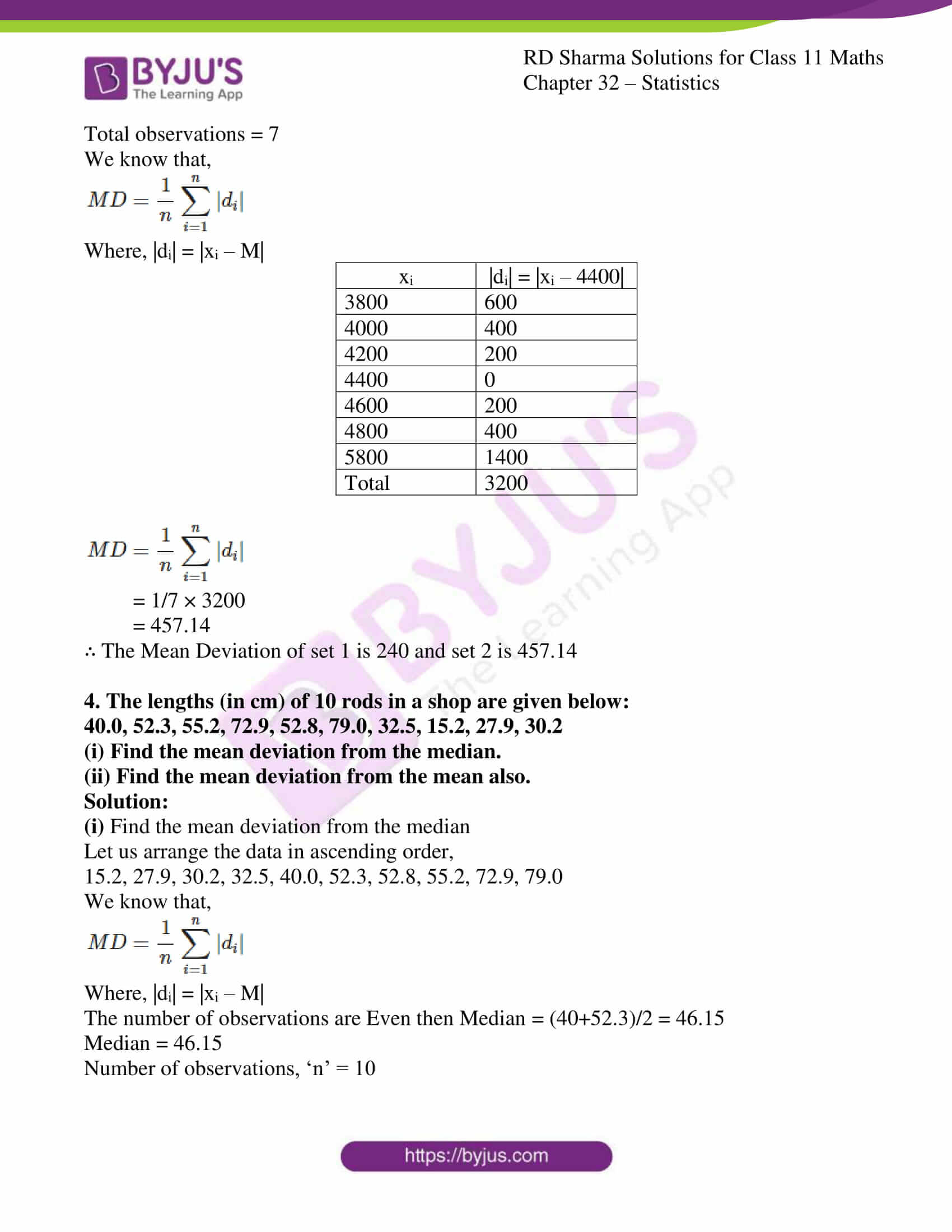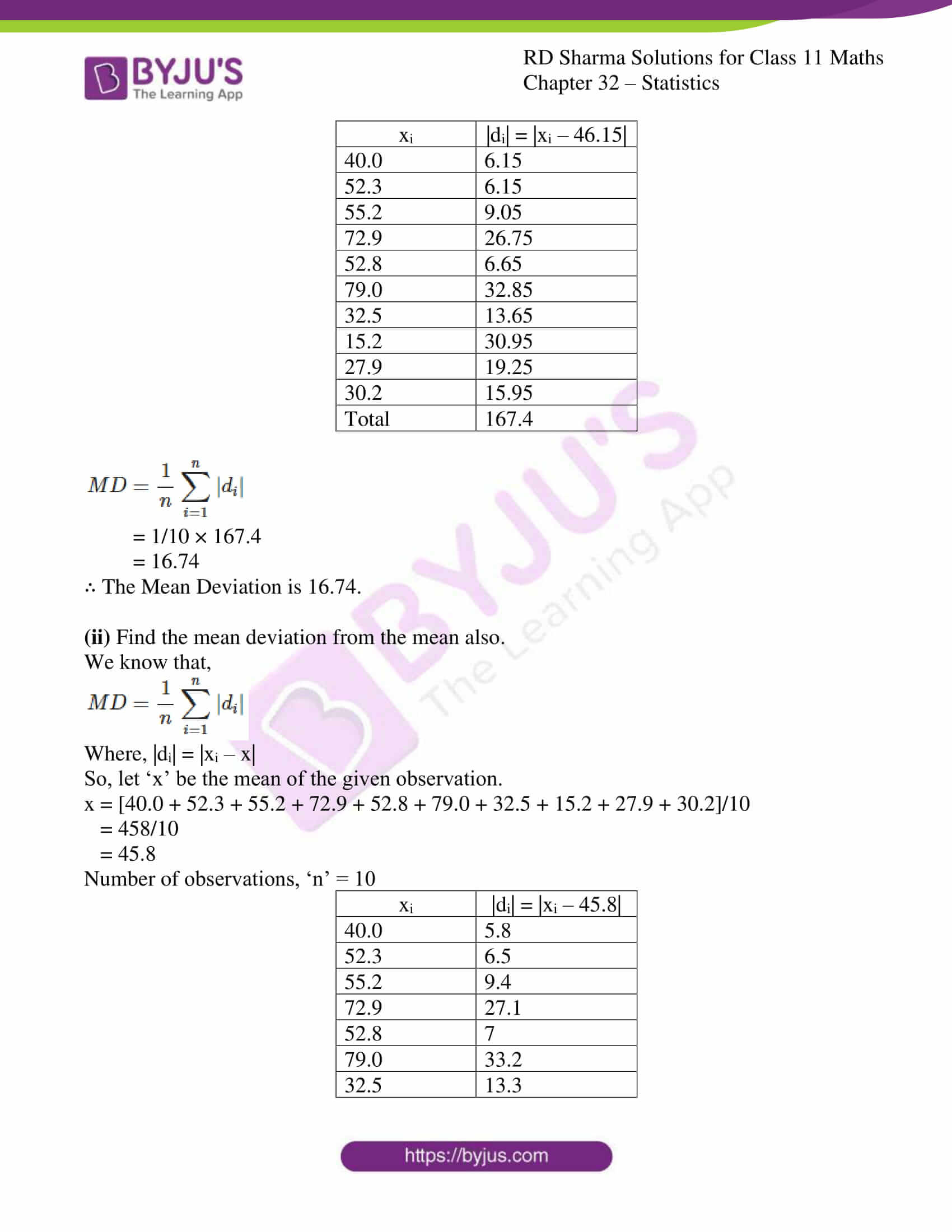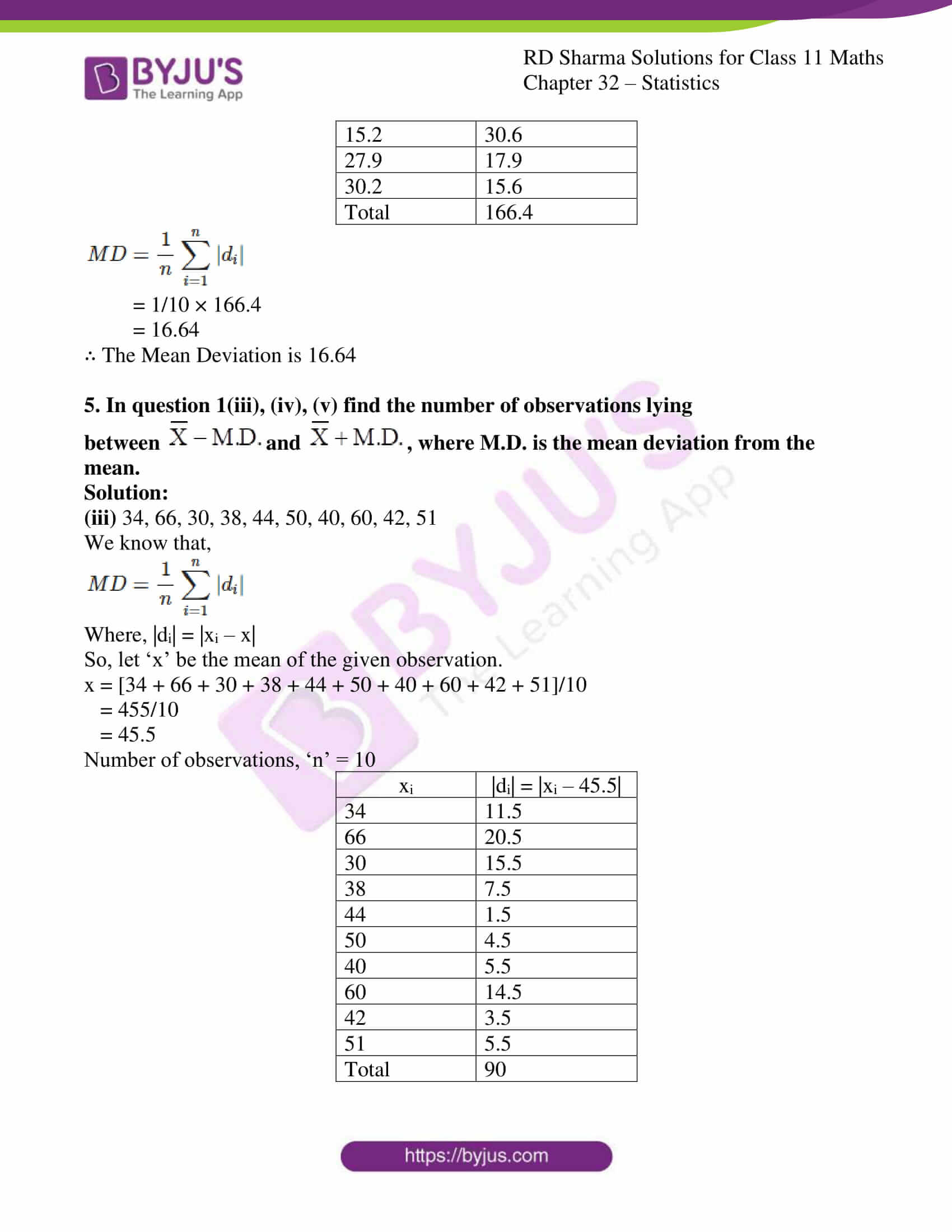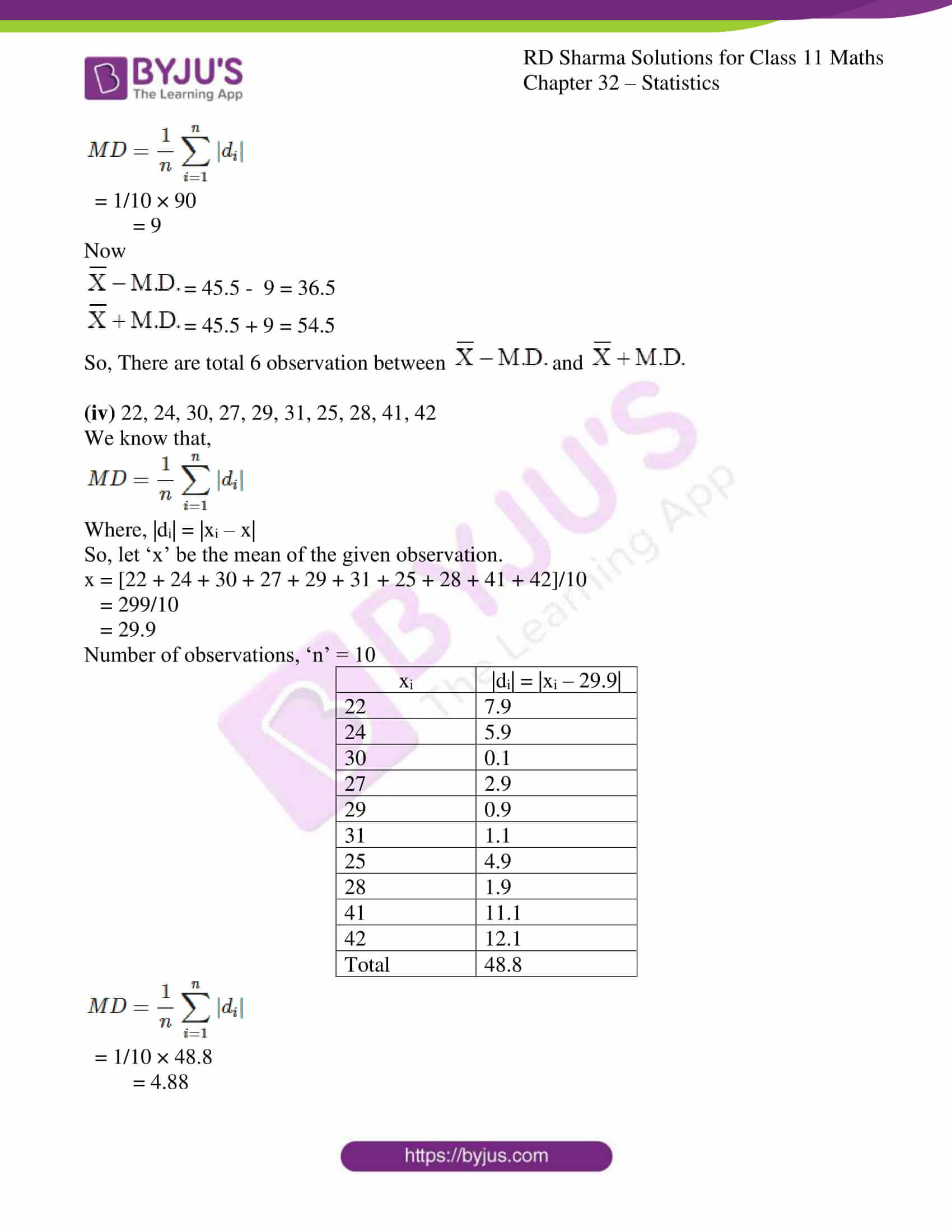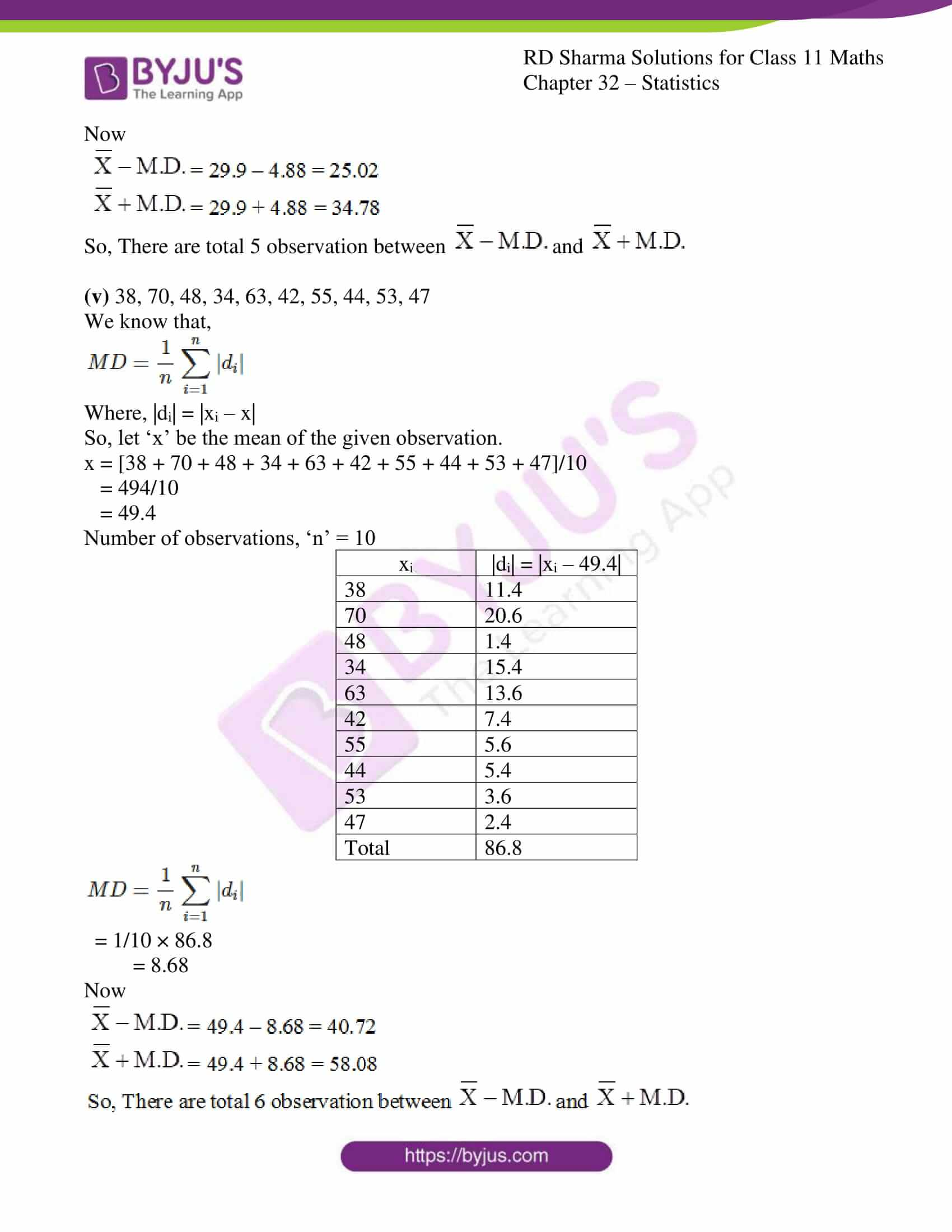### Access answers to RD Sharma Solutions for Class 11 Maths Exercise 32.1 Chapter 32 – Statistics

1. Calculate the mean deviation about the median of the following observation :
(i) 3011, 2780, 3020, 2354, 3541, 4150, 5000

(ii) 38, 70, 48, 34, 42, 55, 63, 46, 54, 44

(iii) 34, 66, 30, 38, 44, 50, 40, 60, 42, 51

(iv) 22, 24, 30, 27, 29, 31, 25, 28, 41, 42

(v) 38, 70, 48, 34, 63, 42, 55, 44, 53, 47

Solution:

(i) 3011, 2780, 3020, 2354, 3541, 4150, 5000

To calculate the Median (M), let us arrange the numbers in ascending order.

Median is the middle number of all the observation.

2354, 2780, 3011, 3020, 3541, 4150, 5000

So, Median = 3020 and n = 7

By using the formula to calculate Mean Deviation,

$$MD = \frac{1}{n}\sum_{i=1}^{n}|d_{i}|$$
 xi |di| = |xi – 3020| 3011 9 2780 240 3020 0 2354 666 3541 521 4150 1130 5000 1980 Total 4546
$$MD = \frac{1}{n}\sum_{i=1}^{n}|d_{i}|$$

= 1/7 × 4546

= 649.42

∴ The Mean Deviation is 649.42.

(ii) 38, 70, 48, 34, 42, 55, 63, 46, 54, 44

To calculate the Median (M), let us arrange the numbers in ascending order.

Median is the middle number of all the observation.

34, 38, 42, 44, 46, 48, 54, 55, 63, 70

Here the Number of observations are Even then Median = (46+48)/2 = 47

Median = 47 and n = 10

By using the formula to calculate Mean Deviation,

$$MD = \frac{1}{n}\sum_{i=1}^{n}|d_{i}|$$
 xi |di| = |xi – 47| 38 9 70 23 48 1 34 13 42 5 55 8 63 16 46 1 54 7 44 3 Total 86
$$MD = \frac{1}{n}\sum_{i=1}^{n}|d_{i}|$$

= 1/10 × 86

= 8.6

∴ The Mean Deviation is 8.6.

(iii) 34, 66, 30, 38, 44, 50, 40, 60, 42, 51

To calculate the Median (M), let us arrange the numbers in ascending order.

Median is the middle number of all the observation.

30, 34, 38, 40, 42, 44, 50, 51, 60, 66

Here the Number of observations are Even then Median = (42+44)/2 = 43

Median = 43 and n = 10

By using the formula to calculate Mean Deviation,

$$MD = \frac{1}{n}\sum_{i=1}^{n}|d_{i}|$$

 xi |di| = |xi – 43| 30 13 34 9 38 5 40 3 42 1 44 1 50 7 51 8 60 17 66 23 Total 87
$$MD = \frac{1}{n}\sum_{i=1}^{n}|d_{i}|$$

= 1/10 × 87

= 8.7

∴ The Mean Deviation is 8.7.

(iv) 22, 24, 30, 27, 29, 31, 25, 28, 41, 42

To calculate the Median (M), let us arrange the numbers in ascending order.

Median is the middle number of all the observation.

22, 24, 25, 27, 28, 29, 30, 31, 41, 42

Here the Number of observations are Even then Median = (28+29)/2 = 28.5

Median = 28.5 and n = 10

By using the formula to calculate Mean Deviation,

$$MD = \frac{1}{n}\sum_{i=1}^{n}|d_{i}|$$
 xi |di| = |xi – 28.5| 22 6.5 24 4.5 30 1.5 27 1.5 29 0.5 31 2.5 25 3.5 28 0.5 41 12.5 42 13.5 Total 47
$$MD = \frac{1}{n}\sum_{i=1}^{n}|d_{i}|$$

= 1/10 × 47

= 4.7

∴ The Mean Deviation is 4.7.

(v) 38, 70, 48, 34, 63, 42, 55, 44, 53, 47

To calculate the Median (M), let us arrange the numbers in ascending order.

Median is the middle number of all the observation.

34, 38, 43, 44, 47, 48, 53, 55, 63, 70

Here the Number of observations are Even then Median = (47+48)/2 = 47.5

Median = 47.5 and n = 10

By using the formula to calculate Mean Deviation,

$$MD = \frac{1}{n}\sum_{i=1}^{n}|d_{i}|$$

 xi |di| = |xi – 47.5| 38 9.5 70 22.5 48 0.5 34 13.5 63 15.5 42 5.5 55 7.5 44 3.5 53 5.5 47 0.5 Total 84
$$MD = \frac{1}{n}\sum_{i=1}^{n}|d_{i}|$$

= 1/10 × 84

= 8.4

∴ The Mean Deviation is 8.4.

2. Calculate the mean deviation from the mean for the following data :
(i) 4, 7, 8, 9, 10, 12, 13, 17

(ii) 13, 17, 16, 14, 11, 13, 10, 16, 11, 18, 12, 17

(iii) 38, 70, 48, 40, 42, 55, 63, 46, 54, 44

(iv) 36, 72, 46, 42, 60, 45, 53, 46, 51, 49
(v) 57, 64, 43, 67, 49, 59, 44, 47, 61, 59

Solution:

(i) 4, 7, 8, 9, 10, 12, 13, 17

We know that,

$$MD = \frac{1}{n}\sum_{i=1}^{n}|d_{i}|$$

Where, |di| = |xi – x|

So, let ‘x’ be the mean of the given observation.

x = [4 + 7 + 8 + 9 + 10 + 12 + 13 + 17]/8

= 80/8

= 10

Number of observations, ‘n’ = 8

 xi |di| = |xi – 10| 4 6 7 3 8 2 9 1 10 0 12 2 13 3 17 7 Total 24
$$MD = \frac{1}{n}\sum_{i=1}^{n}|d_{i}|$$

= 1/8 × 24

= 3

∴ The Mean Deviation is 3.

(ii) 13, 17, 16, 14, 11, 13, 10, 16, 11, 18, 12, 17

We know that,

$$MD = \frac{1}{n}\sum_{i=1}^{n}|d_{i}|$$

Where, |di| = |xi – x|

So, let ‘x’ be the mean of the given observation.

x = [13 + 17 + 16 + 14 + 11 + 13 + 10 + 16 + 11 + 18 + 12 + 17]/12

= 168/12

= 14

Number of observations, ‘n’ = 12

 xi |di| = |xi – 14| 13 1 17 3 16 2 14 0 11 3 13 1 10 4 16 2 11 3 18 4 12 2 17 3 Total 28
$$MD = \frac{1}{n}\sum_{i=1}^{n}|d_{i}|$$

= 1/12 × 28

= 2.33

∴ The Mean Deviation is 2.33.

(iii) 38, 70, 48, 40, 42, 55, 63, 46, 54, 44

We know that,

$$MD = \frac{1}{n}\sum_{i=1}^{n}|d_{i}|$$

Where, |di| = |xi – x|

So, let ‘x’ be the mean of the given observation.

x = [38 + 70 + 48 + 40 + 42 + 55 + 63 + 46 + 54 + 44]/10

= 500/10

= 50

Number of observations, ‘n’ = 10

 xi |di| = |xi – 50| 38 12 70 20 48 2 40 10 42 8 55 5 63 13 46 4 54 4 44 6 Total 84
$$MD = \frac{1}{n}\sum_{i=1}^{n}|d_{i}|$$

= 1/10 × 84

= 8.4

∴ The Mean Deviation is 8.4.

(iv) 36, 72, 46, 42, 60, 45, 53, 46, 51, 49

We know that,

$$MD = \frac{1}{n}\sum_{i=1}^{n}|d_{i}|$$

Where, |di| = |xi – x|

So, let ‘x’ be the mean of the given observation.

x = [36 + 72 + 46 + 42 + 60 + 45 + 53 + 46 + 51 + 49]/10

= 500/10

= 50

Number of observations, ‘n’ = 10

 xi |di| = |xi – 50| 36 14 72 22 46 4 42 8 60 10 45 5 53 3 46 4 51 1 49 1 Total 72
$$MD = \frac{1}{n}\sum_{i=1}^{n}|d_{i}|$$

= 1/10 × 72

= 7.2

∴ The Mean Deviation is 7.2.

(v) 57, 64, 43, 67, 49, 59, 44, 47, 61, 59

We know that,

$$MD = \frac{1}{n}\sum_{i=1}^{n}|d_{i}|$$

Where, |di| = |xi – x|

So, let ‘x’ be the mean of the given observation.

x = [57 + 64 + 43 + 67 + 49 + 59 + 44 + 47 + 61 + 59]/10

= 550/10

= 55

Number of observations, ‘n’ = 10

 xi |di| = |xi – 55| 57 2 64 9 43 12 67 12 49 6 59 4 44 11 47 8 61 6 59 4 Total 74
$$MD = \frac{1}{n}\sum_{i=1}^{n}|d_{i}|$$

= 1/10 × 74

= 7.4

∴ The Mean Deviation is 7.4.

3. Calculate the mean deviation of the following income groups of five and seven members from their medians:

 I Income in ₹ II Income in ₹ 4000 3800 4200 4000 4400 4200 4600 4400 4800 4600 4800 5800

Solution:

Let us calculate the mean deviation for the first data set.

Since the data is arranged in ascending order,

4000, 4200, 4400, 4600, 4800

Median = 4400

Total observations = 5

We know that,

$$MD = \frac{1}{n}\sum_{i=1}^{n}|d_{i}|$$

Where, |di| = |xi – M|

 xi |di| = |xi – 4400| 4000 400 4200 200 4400 0 4600 200 4800 400 Total 1200
$$MD = \frac{1}{n}\sum_{i=1}^{n}|d_{i}|$$

= 1/5 × 1200

= 240

Let us calculate the mean deviation for the second data set.

Since the data is arranged in ascending order,

3800, 4000, 4200, 4400, 4600, 4800, 5800

Median = 4400

Total observations = 7

We know that,

$$MD = \frac{1}{n}\sum_{i=1}^{n}|d_{i}|$$

Where, |di| = |xi – M|

 xi |di| = |xi – 4400| 3800 600 4000 400 4200 200 4400 0 4600 200 4800 400 5800 1400 Total 3200
$$MD = \frac{1}{n}\sum_{i=1}^{n}|d_{i}|$$

= 1/7 × 3200

= 457.14

∴ The Mean Deviation of set 1 is 240 and set 2 is 457.14

4. The lengths (in cm) of 10 rods in a shop are given below:
40.0, 52.3, 55.2, 72.9, 52.8, 79.0, 32.5, 15.2, 27.9, 30.2
(i) Find the mean deviation from the median.
(ii) Find the mean deviation from the mean also.

Solution:

(i) Find the mean deviation from the median

Let us arrange the data in ascending order,

15.2, 27.9, 30.2, 32.5, 40.0, 52.3, 52.8, 55.2, 72.9, 79.0

We know that,

$$MD = \frac{1}{n}\sum_{i=1}^{n}|d_{i}|$$

Where, |di| = |xi – M|

The number of observations are Even then Median = (40+52.3)/2 = 46.15

Median = 46.15

Number of observations, ‘n’ = 10

 xi |di| = |xi – 46.15| 40.0 6.15 52.3 6.15 55.2 9.05 72.9 26.75 52.8 6.65 79.0 32.85 32.5 13.65 15.2 30.95 27.9 19.25 30.2 15.95 Total 167.4
$$MD = \frac{1}{n}\sum_{i=1}^{n}|d_{i}|$$

= 1/10 × 167.4

= 16.74

∴ The Mean Deviation is 16.74.

(ii) Find the mean deviation from the mean also.

We know that,

$$MD = \frac{1}{n}\sum_{i=1}^{n}|d_{i}|$$

Where, |di| = |xi – x|

So, let ‘x’ be the mean of the given observation.

x = [40.0 + 52.3 + 55.2 + 72.9 + 52.8 + 79.0 + 32.5 + 15.2 + 27.9 + 30.2]/10

= 458/10

= 45.8

Number of observations, ‘n’ = 10

 xi |di| = |xi – 45.8| 40.0 5.8 52.3 6.5 55.2 9.4 72.9 27.1 52.8 7 79.0 33.2 32.5 13.3 15.2 30.6 27.9 17.9 30.2 15.6 Total 166.4
$$MD = \frac{1}{n}\sum_{i=1}^{n}|d_{i}|$$

= 1/10 × 166.4

= 16.64

∴ The Mean Deviation is 16.64

5. In question 1(iii), (iv), (v) find the number of observations lying between $$\overline{X} – M.D$$ and $$\overline{X} + M.D$$, where M.D. is the mean deviation from the mean.

Solution:

(iii) 34, 66, 30, 38, 44, 50, 40, 60, 42, 51

We know that,

$$MD = \frac{1}{n}\sum_{i=1}^{n}|d_{i}|$$

Where, |di| = |xi – x|

So, let ‘x’ be the mean of the given observation.

x = [34 + 66 + 30 + 38 + 44 + 50 + 40 + 60 + 42 + 51]/10

= 455/10

= 45.5

Number of observations, ‘n’ = 10

 xi |di| = |xi – 45.5| 34 11.5 66 20.5 30 15.5 38 7.5 44 1.5 50 4.5 40 5.5 60 14.5 42 3.5 51 5.5 Total 90
$$MD = \frac{1}{n}\sum_{i=1}^{n}|d_{i}|$$

= 1/10 × 90

= 9

Now

$$\overline{X} – M.D$$ = 45.5 – 9 = 36.5

$$\overline{X} + M.D$$ = 45.5 + 9 = 54.5

So, There are total 6 observation between $$\overline{X} – M.D$$ and $$\overline{X} + M.D$$

(iv) 22, 24, 30, 27, 29, 31, 25, 28, 41, 42

We know that,

$$MD = \frac{1}{n}\sum_{i=1}^{n}|d_{i}|$$

Where, |di| = |xi – x|

So, let ‘x’ be the mean of the given observation.

x = [22 + 24 + 30 + 27 + 29 + 31 + 25 + 28 + 41 + 42]/10

= 299/10

= 29.9

Number of observations, ‘n’ = 10

 xi |di| = |xi – 29.9| 22 7.9 24 5.9 30 0.1 27 2.9 29 0.9 31 1.1 25 4.9 28 1.9 41 11.1 42 12.1 Total 48.8
$$MD = \frac{1}{n}\sum_{i=1}^{n}|d_{i}|$$

= 1/10 × 48.8

= 4.88

Now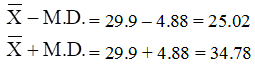So, There are total 5 observation between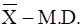and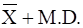(v) 38, 70, 48, 34, 63, 42, 55, 44, 53, 47

We know that,

$$MD = \frac{1}{n}\sum_{i=1}^{n}|d_{i}|$$

Where, |di| = |xi – x|

So, let ‘x’ be the mean of the given observation.

x = [38 + 70 + 48 + 34 + 63 + 42 + 55 + 44 + 53 + 47]/10

= 494/10

= 49.4

Number of observations, ‘n’ = 10

 xi |di| = |xi – 49.4| 38 11.4 70 20.6 48 1.4 34 15.4 63 13.6 42 7.4 55 5.6 44 5.4 53 3.6 47 2.4 Total 86.8
$$MD = \frac{1}{n}\sum_{i=1}^{n}|d_{i}|$$

= 1/10 × 86.8

= 8.68

Now